# Count Cells Equal to one of two Results – Excel & Google Sheets

In this tutorial, we will count the number of cells that contain either one result or another result.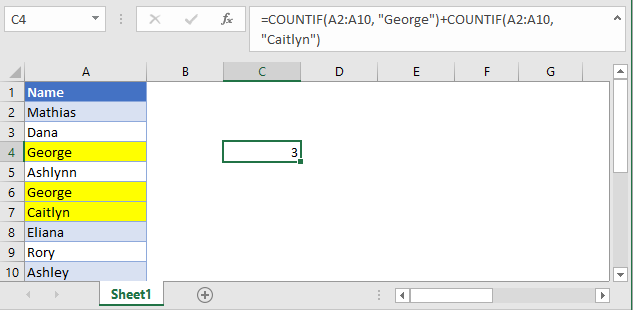## Count Cells Equal to with COUNTIF

The COUNTIF function counts the number of times the specified value exists in the range. By adding together the results of two COUNTIF functions we can calculate the number of items that are equal to one of two results.

Using the data set above, let’s say that we want to find the number of times the name “George” and the name “Caitlyn” exists.

``=COUNTIF(A2:A10, “George”) + COUNTIF(A2:A10, “Caitlyn”)``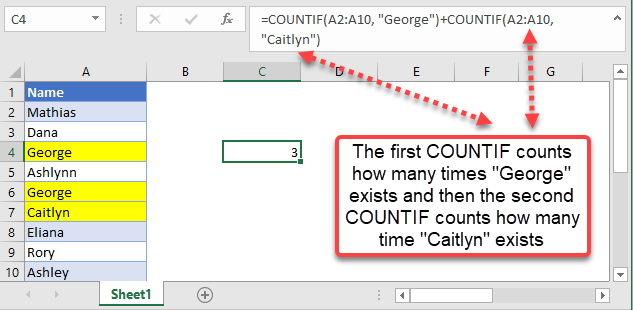We end up with a result of 3. This is because the name “George” exists twice in that range (in cells A4 and A6) and the name “Caitlyn” exists only once (in cell A7).

Please keep in mind that if you can look for as many results as you would like, just remember to add the additional “+” sign.

You can also use wildcards to find partial matches. For example, if you want to count the number of cells that start with G and the number of cells that start with A, we can use the following formula.

``=COUNTIF(A2:A10, “G*") + COUNTIF(A2:A10, “A*”)``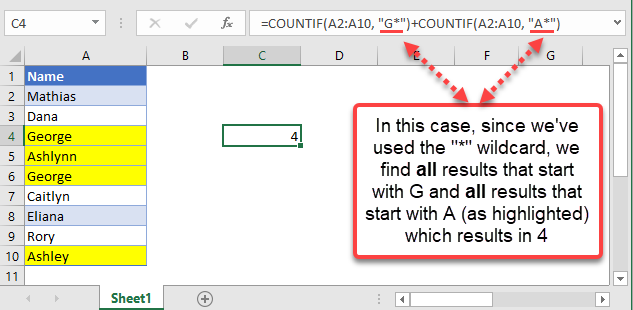This results in 4 because we have two names starting with G (A4 and A6) and two names that start with C (A7 and A11).

## Count Cells Equal to X or Y in Google Sheets

The above functions work the same way in Google Sheets as well.

``=COUNTIF(A2:A10, “George”) + COUNTIF(A2:A10, “Caitlyn”)``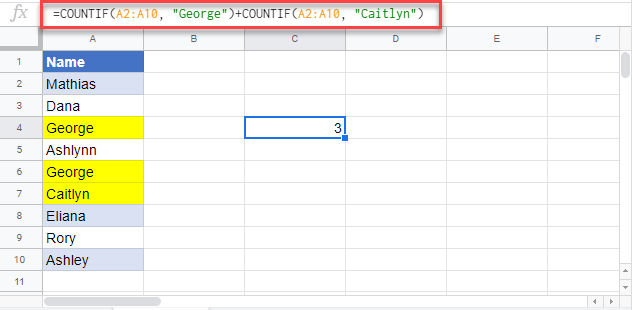``=COUNTIF(A2:A10, “G*") + COUNTIF(A2:A10, “A*”)``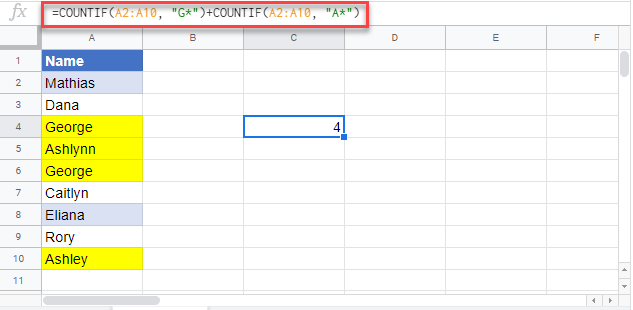### Excel Practice Worksheet

Practice Excel functions and formulas with our 100% free practice worksheets!